69 篇文章 1 订阅

哈希概念

• 计算元素key在数组中的位置为index

• key插入到index位置即可

index=key%array.length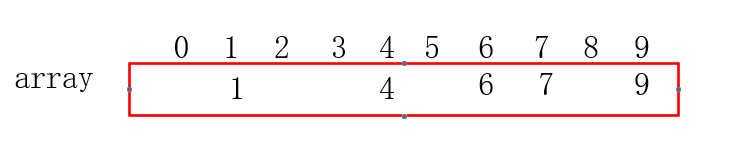• 计算元素x可能在数组中的位置index（有可能查找的元素不存在）
• index位置取元素即可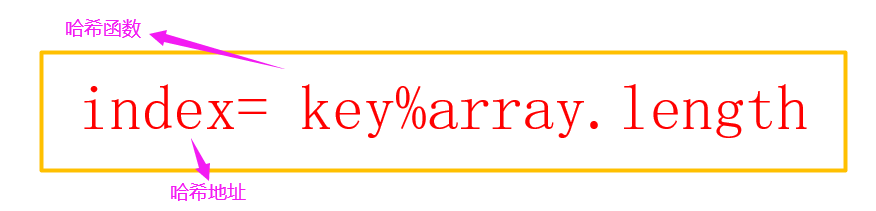常见的哈希函数

• 直接定制法

• 除留余数法（常用）

Hash(key) = key% pp一般选用质数；

• 平方取中法

• 折叠法

• 随机数法

H(key) = random(key),其中random为随机数函数。

• 数学分析法

哈希冲突

• 哈希函数的定义域必须包括需要存储的全部关键码；
• 哈希函数计算出来的地址能均匀分布在整个空间中；
• 哈希函数设计应该尽比较简单；

解决冲突的两种主要解决方式

方式一：闭散列

• 线性探测
• 二次探测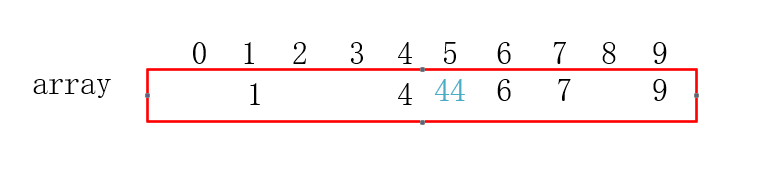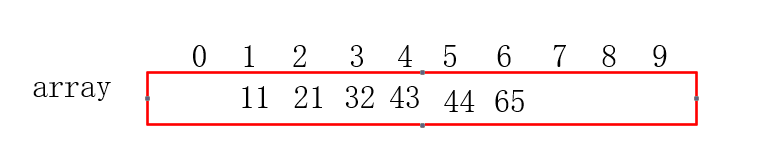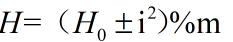H0为通过哈希函数计算出的散列地址，m为哈希表的容量大小，i为自然数：1，2，3…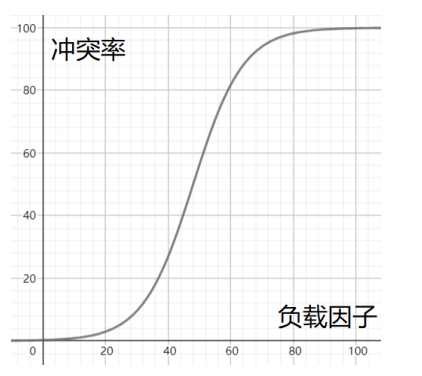方式二：开散列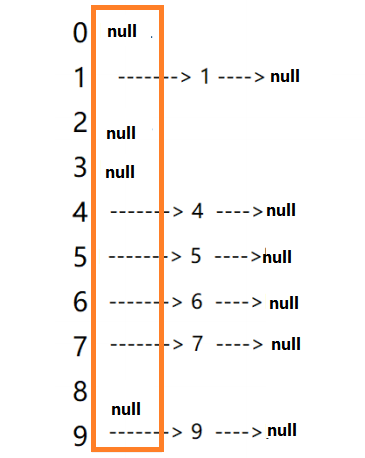简单实现哈希桶

package day20211128;

public class MyHashBucket {
//定义结点
public static class  ListNode{
Integer key;
Integer value;
ListNode next;  //下一个引用

public ListNode(Integer key,Integer value){
this.key=key;
this.value=value;

}
}
ListNode[] table;   //用来存放链表的数组
int size; //哈希桶中有效元素的个数

public MyHashBucket(int initCapacity){
initCapacity= initCapacity <= 0?16:initCapacity;
table=new ListNode[initCapacity];
}

//插入---->put
public Integer put(Integer key,Integer value){
checkCapacity();
//1.根据哈希函数计算对应的桶号
int bucketNum=hashFunc(key);
//检测所插入元素的key在哈希桶中是否已经存在----key是唯一的
//相当于在单链表中查找一个元素是否存在
ListNode cur=table[bucketNum];
while(cur!=null){
if(key.equals(cur.key)){
//key存在时，将旧值进行替换
Integer oldValue=cur.value;
cur.value=value;
return oldValue;
}
cur=cur.next;
}
//key不存在时
//2.将元素插入到哈希桶(bucketNum)中
ListNode newNode=new ListNode(key, value);
newNode.next=table[bucketNum];
table[bucketNum]=newNode;
size++;
return value;
}

public Integer get (Integer key){
//1.根据哈希函数计算对应的桶号
int bucketNum=hashFunc(key);

//2.到桶中找key
ListNode cur=table[bucketNum];
while(cur!=null){
if(key.equals(cur.key)){
return cur.value;
}
}
return null;
}

public Integer getOrDefault(Integer key,Integer value){
Integer ret=get(key);
if(ret!=null){
return ret;
}
return value;
}

//删除--->remove
public  Integer remove(Integer key){
//通过哈希函数计算对应的桶号
int bucketNum=hashFunc(key);

//在桶(bucketNum)中找要删除的结点
ListNode cur=table[bucketNum];
ListNode prev=null;
while(cur!=null){
if(key.equals(cur.key)){
Integer oldValue=cur.value;
//删除的结点刚好是第一个
if(table[bucketNum]==cur){
table[bucketNum]=cur.next;
//cur.next=null;
}else{
//删除其他结点
prev.next=cur.next;
//cur.next=null;
}
cur.next=null;
size--;
return  oldValue;
}

cur=cur.next;

}
return  null;
}

public boolean containsKey(Integer key){
//根据哈希的特性来找
return  null!=get(key);
}

public boolean containsValue(Integer value){
//每个桶进行遍历找
for(int bucketNum=0;bucketNum<table.length;bucketNum++){
ListNode cur=table[bucketNum];
while (cur!=null){
if(value.equals(cur.value)){
return  true;
}
cur=cur.next;
}
}
return false;
}
public int size(){
return size;
}

private void checkCapacity(){
if(size>=table.length){
int newCapacity=table.length*2;
ListNode[] newTable=new ListNode[newCapacity];

//将就数组table中的元素搬移到扩容之后的newTable中
//每个结点都需要搬移
for(int i=0;i<table.length;i++){
//逐个桶进行搬移
ListNode cur=table[i];
while (cur!=null){
//将cur先从tablet中移除
table[i]=cur.next;

//将移除的cur往newTablet中插入
int bucketNum=cur.key % newTable.length;
cur.next=newTable[bucketNum];
newTable[bucketNum]=cur;
cur=table[i];
}
}
table=newTable;
}

}
private Integer hashFunc(Integer key){
return key % table.length;

}
}

• 哈希函数采用的是除留余数法，但只是针对key为整形的情况，当key不是整形时，就不能用了；
• 扩容时，没有考虑负载因子的调节，直接将负载因子设为1了；
• 虽然通过扩容可以将一部分较长的链表缓解，但随着插入元素的不断增多，查找的效率就下降了；

和Java类集的关系

• Java中利用哈希表实现MapSet
• Java中使用的是哈希桶方式解决冲突的
• Java 中，当发生冲突链表长度大于一定阈值后，将链表转变为搜索树（红黑树）
• Java中计算哈希地址值实际上是调用的是类的 hashCode（) 方法,进行 key 的相等的比较调用的是 equals 方法

带你看标准库中关于HashMap的设计

1.HashMap的默认初始容量是16

/**
* The default initial capacity - MUST be a power of two.
*/
static final int DEFAULT_INITIAL_CAPACITY = 1 << 4; // aka 16

2.HashMap的最大容量为2的30次方

/**
* The maximum capacity, used if a higher value is implicitly specified
* by either of the constructors with arguments.
* MUST be a power of two <= 1<<30.
*/
static final int MAXIMUM_CAPACITY = 1 << 30;

3.HashMap的默认负载因子是0.75

/**
* The load factor used when none specified in constructor.
*/
static final float DEFAULT_LOAD_FACTOR = 0.75f;

4.链表与红黑树相互转化

/**
* The bin count threshold for using a tree rather than list for a
* bin.  Bins are converted to trees when adding an element to a
* bin with at least this many nodes. The value must be greater
* than 2 and should be at least 8 to mesh with assumptions in
* tree removal about conversion back to plain bins upon
* shrinkage.
*/若链表中节点个数超过8时，会将链表转化为红黑树
static final int TREEIFY_THRESHOLD = 8;

/**
* The bin count threshold for untreeifying a (split) bin during a
* resize operation. Should be less than TREEIFY_THRESHOLD, and at
* most 6 to mesh with shrinkage detection under removal.
/// 若红黑树中节点小于6时，红黑树退还为链表
static final int UNTREEIFY_THRESHOLD = 6;
/**
* The smallest table capacity for which bins may be treeified.
* (Otherwise the table is resized if too many nodes in a bin.)
* Should be at least 4 * TREEIFY_THRESHOLD to avoid conflicts
* between resizing and treeification thresholds.
*/如果哈希桶中某条链表的个数超过8，并且桶的个数超过64时才会将链表转换为红黑树，否则直接扩容
static final int MIN_TREEIFY_CAPACITY = 64;

5.哈希表的容量转换为2的幂次方

static final int tableSizeFor(int cap) {
int n = cap - 1;
n |= n >>> 1;
n |= n >>> 2;
n |= n >>> 4;
n |= n >>> 8;
n |= n >>> 16;
return (n < 0) ? 1 : (n >= MAXIMUM_CAPACITY) ? MAXIMUM_CAPACITY : n + 1;
}

6.将key转换为整形数字

static final int hash(Object key) {
int h;
return (key == null) ? 0 : (h = key.hashCode()) ^ (h >>> 16);
}

Java8对于数组table的初始化，并没有直接放在构造器中完成，而是将table数组的构造延迟到了（resize）扩容中完成；

HashMap常考问题

• HashMap何时开辟bucket数组占用内存？

• HashMap为什么要重写HashCode与equals？

HashMap是泛型的，里面存放的是K-V键值对，而它的哈希函数实质采用的是除留余数法，因此，当key不是整形时，就需要用户提供HashCode方法，将key转为整形；

• HashMap何时扩容？

• new HashMap（19），bucket数组多大？

08-28130
05-276905-17714
08-1570
08-2358
11-242257
07-2226
03-16230
04-23168
07-13576
10-02142
09-09147
06-1797
06-1569
09-1371
02-21225
03-1829
12-17282
03-2836
06-16173Java小菜鸟～

¥2 ¥4 ¥6 ¥10 ¥20余额支付 (余额：-- )扫码支付获取中扫码支付点击重新获取扫码支付1.余额是钱包充值的虚拟货币，按照1:1的比例进行支付金额的抵扣。
2.余额无法直接购买下载，可以购买VIP、C币套餐、付费专栏及课程。余额充值